Home > CCA2 > Chapter 8 > Lesson 8.3.2 > Problem8-149

8-149.
1. Rewrite each of the following division problems as a single complex number. Homework Help ✎

1.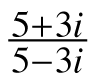2.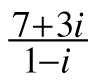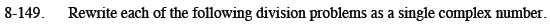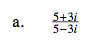Multiply by a Giant One using the conjugate of the denominator.

$\frac{5 + 3i}{5-3i} \cdot \frac{5 + 3i}{5+ 3i}$

$\frac{(5+3i)(5+3i)}{(5-3i)(5+3i)} =\frac{16 + 30i}{34}$

$\frac{8}{17} + \frac{15}{17}i$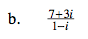See part (a).